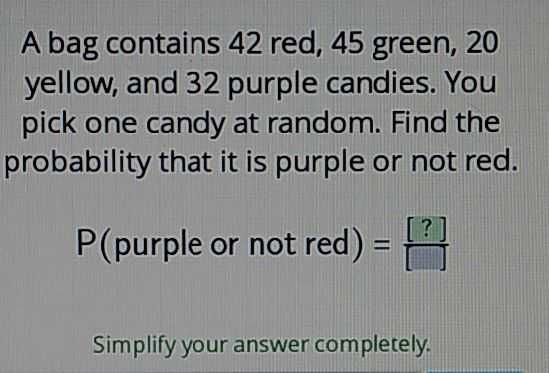### ¿Todavía tienes preguntas de matemáticas?

Pregunte a nuestros tutores expertos
Algebra
PreguntaA bag contains $$42$$ red, $$45$$ green, $$20$$ yellow, and $$32$$ purple candies. You pick one candy at random. Find the probability that it is purple or not red.

$$P$$ (purple or not red) $$= \frac { [ ? ] } { \square }$$

$$\frac{32}{139}$$